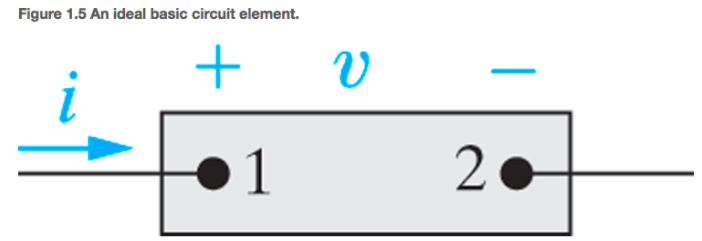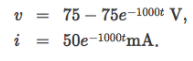# Problem: The voltage and current at the terminals of the circuit element in Fig 1.5 are zero for t &lt; 0. For t &gt; 0 they area) Find the maximum value of the power delivered to the circuit.b) Find the total energy delivered to the element

###### FREE Expert Solution

Power in the circuit is expressed as:

$\overline{){\mathbf{P}}{\mathbf{=}}{\mathbf{V}}{\mathbf{i}}}$, where V is voltage and i is current.

Total energy delivered to the element is found from:

$\overline{){\mathbf{w}}{\mathbf{=}}{{\mathbf{\int }}}_{{\mathbf{0}}}^{{\mathbf{\infty }}}{\mathbf{p}}{\mathbf{\left(}}{\mathbf{t}}{\mathbf{\right)}}{\mathbf{d}}{\mathbf{t}}}$

a)

From the equation for power:

$\begin{array}{rcl}\mathbf{P}& \mathbf{=}& \mathbf{V}\mathbf{i}\\ & \mathbf{=}& \mathbf{\left(}\mathbf{75}\mathbf{-}\mathbf{75}{\mathbf{e}}^{\mathbf{-}\mathbf{1000}\mathbf{t}}\mathbf{\right)}\mathbf{\left(}\mathbf{50}{\mathbf{e}}^{\mathbf{-}\mathbf{1000}\mathbf{t}}\mathbf{×}{\mathbf{10}}^{\mathbf{-}\mathbf{3}}\mathbf{\right)}\\ & \mathbf{=}& \mathbf{3}\mathbf{.}\mathbf{75}{\mathbf{e}}^{\mathbf{-}\mathbf{1000}\mathbf{t}}\mathbf{-}\mathbf{3}\mathbf{.}\mathbf{75}{\mathbf{e}}^{\mathbf{-}\mathbf{2000}\mathbf{t}}\end{array}$

93% (403 ratings)###### Problem Details

The voltage and current at the terminals of the circuit element in Fig 1.5 are zerofor t < 0. For t > 0 they area) Find the maximum value of the power delivered to the circuit.

b) Find the total energy delivered to the element